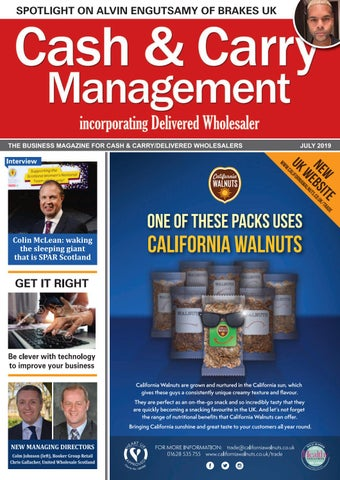TH

BE T O N N S A N C O NT EAS E M R E L S I A T R EG E L V R D O A F IS

N

W O H S

TH

BE T O N N S A N C O NT EAS E M R E L S I A T R EG E L V R D O A F IS

N

W O H S

• The Netherlands’ • A brand with over of •

, by popular demand